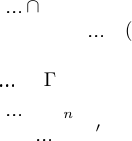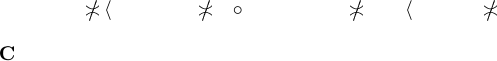Algebraic General Topology. Vol 1: Paperback / E-book || Axiomatic Theory of Formulas: Paperback / E-book

As an Amazon Associate I earn from qualifying purchases.

Distributivity of co mposition with a principal reloid
over join of reloids
by Victor Porton
Email: porton@narod.ru
Web: http://www.mathematics21.org
September 23, 2013
1 Introduction
It is a draft.
I present a proof of the equation (
F
T ) F =
F
{G F | G T } for a principal reloid F and a
set T of reloids (provided their sources and destination match each other).
First read my boo k .
1.1 Decomposition of composition of binary relations
Remark 1. Sorry for unfortunate choice of termino logy: “composition” and “decomposisiton” are
unrelated.
The idea of the proof below is that composition of binary relations can be decomposed into two
operations: and dom:
g f = {((x; z); y) | xfy ygz}.
Composition of binary relations can be decomposed: g f = dom(g f).
It ca n be decomposed even further: g f = Θ
0
f Θ
1
g where
Θ
0
f = {((x; z) ; y) | xfy, z } and Θ
1
f = {((x; z); y) | yfz, x }.
(Here is the Grothendieck universe.)
Now we will do a similar tr ick with reloids.
1.2 Decomposition of composition of reloids
A similar thing for reloids:
g f =
l
RLD(Src f ;Dst g)
(G F ) | F GR f , G GR g
=
l
RLD(Src f ;Dst g)
dom(G F ) | F
GR f , G GR g
.
Lemma 2. {G F | F f , G g} is a ﬁlter base.
Proof. Let P , Q {G F | F f , G g}. Then P = G
0
F
0
, Q = G
1
F
1
for some F
0
, F
1
f ,
G
0
, G
1
g. Then F
0
F
1
f, G
0
G
1
g and thus
P Q (F
0
F
1
) (G
0
G
1
) {G F | F f , G g }.
Corollary 3.
RLD(Src f ×Dst g;)
(G F ) | F GR f , G GR g
is a generalized ﬁlter base.
Propositio n 4. g f = dom
d
RLD(Src f ×Dst g;)
(G F ) | F GR f , G GR g
.
Proof.
RLD(Src f ;Dst g)
dom(G F ) dom
d
RLD(Src f ×Dst g;)
(G F ) | F GR f , G GR g
.
Thus
g f dom
l
RLD(Src f ×Dst g;)
(G F ) | F GR f , G GR g
.
1Let X dom
d
RLD(Src f ×Dst g;)
(G F ) | F GR f , G GR g
. Then there exist Y such that
X × Y
d
RLD(Src f ×Dst g;)
(G F ) | F GR f , G GR g
. So because it is a generalized
ﬁlter base X × Y G F for some F GR f, G GR g. Thus X dom(G F ), X
RLD(Src f ;Dst g)
dom(G F ), X GR(g f).
We can deﬁne g f for reloids f , g:
g f = {G F | F GR f , G GR g}.
Then
g f =
l
RLD(Src f ;Dst g)
hdomi(g f ) = dom
l
RLD(Src f ×Dst g;)
(g f ).
2 Lemmas for the main resu lt
Let F =
RLD(Src F ;Dst F )
f is a principal reloid.
Lemma 5. (g f) (h f) = (g h) f for binary relations f , g, h.
Proof. (g h) f = Θ
0
f Θ
1
(g h) = Θ
0
f
1
g Θ
1
h) =
0
f Θ
1
g)
0
f Θ
1
h) =
(g f) (h f).
Lemma 6.
d
RLD(Src f ×Dst g;)
(G f ) | G
F
T
=
F
d
RLD(Src f ×Dst g;)
(G F ) | G
T
.
Proof.
d
RLD(Src f ×Dst g;)
(G f ) | G GR
F
T
F
d
RLD(Src f ×Dst g;)
(G F ) | G
T
is obvious.
Let K
F
d
RLD(Src f ×Dst g;)
(G F ) | G T
. Then for each G T
K
l
RLD(Src f ×Dst g;)
(G F );
K
d
RLD(Src f ×Dst g;)
{Γ f | Γ GR G}.
K {
0
f )
n
f ) | n N, Γ
i
GR G}.
G T : K
G,0
f )
G,n
f ) for some n N, Γ
G,i
G.
Let G
T
T .
K
0
f )
n
f ) where Γ
i
=
S
gG
Γ
g,i
GR
F
T .
K {
0
f )
n
f ) | n N}.
So K {
0
f )
n
f ) | n N, Γ
i
GR
F
T } =
d
RLD(Src f ×Dst g;)
{G f | G
GR
F
T }.
3 Proof of the main result
Theorem 7. (
F
T ) F =
F
{G F | G T } for every prin cipal reloid F =
RLD(Src f ;Dst g)
f.
Proof.
G
T
F =
l
RLD(Src f ;Dst g)
hdomi
G
T
F
= dom
l
RLD(Src f ×Dst g;)

G
T
F
= dom
l
RLD(Src f ×Dst g;)
G f | G GR
G
T
G
{G F | G T } =
G
l
RLD(Src f ;Dst g)
hdomi(G F ) | G T
=
G
dom
l
RLD(Src f ×Dst g;)
(G F ) | G T
= dom
G
l
RLD(Src f ×Dst g;)
(G F ) | G T
.
2 Section 3It’s enough to prove
l
RLD(Src f ×Dst g;)
(G f ) | G GR
G
T
=
G
l
RLD(Src f ×Dst g;)
(G F ) | G T
but this is the statement of the lemma.
4 Embedding reloids into funcoids
Deﬁnition 8. Let f is a reloid. The funcoid ρf FCD(Src f; Dst f ) is deﬁned by the formulas:
hρf ix = f x and hρ f
1
iy = f
1
y
where x are endo-reloids on Src f a nd y are endo-reloids on Dst f .
Propositio n 9. It is really a funcoid (if we equate reloids x and y with corresponding ﬁlters on
cartesian products of sets).
Proof. y
hρf ix y
f x f
1
y
x hρ f
1
iy
x.
Corollary 10. (ρf )
1
= ρ f
1
.
Deﬁnition 11. It can be continued to arbitrary funcoi ds x having source Src f by the formula
hρ
f ix = hρf iid
Src f
x.
Propositio n 12. ρ is an injection.
Proof. Consider x = id
Src f
.
Propositio n 13. ρ(g f) = (ρg) (ρf ).
Proof. hρ(g f )ix = g f x = hρgihρf ix = (hρ gi hρf i)x. Thus hρ(g f)i = hρgi hρf i =
h(ρg) (ρf)i and so ρ(g f) = (ρg) (ρf).
Theorem 14. ρ
F
F =
F
hρiF f or a set F of reloids.
Proof. It’s enough to prove hρ
F
F i
X = h
F
hρiF i
X for a set X.
Really, hρ
F
F i
X = hρ
F
F i↑X =
F
F X =
F
{f X | f F } =
F
{hρf i↑X | f F } =
h
F
{ρf | f F }i↑X = h
F
hρiF i
X.
Conjecture 1 5. ρ
d
F =
d
hρiF f or a set F of reloids.
Propositio n 16. ρid
RLD(A)
= id
FCD(A)
.
Proof.
ρid
RLD(A)
x = id
RLD(A)
x = x.
We can try to develop further theory by applying embedding of rel oids into funcoids for
researching of properties of reloids.
Theorem 17. Reloid f is monovalued iﬀ funcoid ρf is monovalued.
Proof. ρf is monovalued(ρf) (ρf )
1
1
Dst ρf
ρ(f f
1
) 1
Dst f
ρ(f f
1
) id
RLD(A)
ρ(f f
1
) ρid
FCD(A)
f f
1
id
FCD(A)
f is monovalued.
Bib liogr aphy
 Victor Porton. Algebraic General Topology. Volume 1. 2013.
Bibliography 3